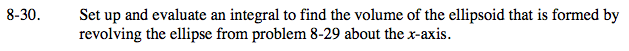### Home > CALC > Chapter 8 > Lesson 8.1.3 > Problem8-30

8-30.$\text{Volume of revolution (disk method)}=\pi \int_{a}^{b}(\text{radius})^{2}dx=\pi \int_{a}^{b}(f(x))^{2}dx$

where f(x) indicates a function.

Obviously, an ellipse is NOT a functions. So you cannot apply the volume of revolution formula above to the entire equation of the ellipse. Thus, when identifying the rotated 'flag', it is necessary to consider only the top or only the bottom half of the ellipse.